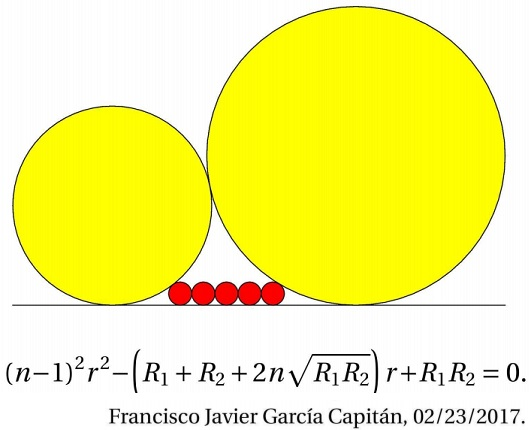# FJG Capitán's Sangaku

### Source### Solution

Assume that the left yellow circle is described by $x^2+(y-R_1)^2=R_1^2;\,$ the right yellow circle by $(x-a)^2+(y-R_2)^2=R_2^2,\,$ $a\gt 0.\,$ The two being externally tangent, we have by the Pythagorean theorem,

$a^2+(R_1-R_2)^2=(R_1+R_2)^2,$

implying $a=2\sqrt{R_1R_2}.\,$ Hence, the right yellow circle is described by $(x-2\sqrt{R_1R_2})^2+(y-R_2)^2=R_2^2.\,$

The rightmost red circle is described by $(x-b)^2+(y-r)^2=r^2.\,$ It being externally tangent to the right yellow circle, we get $(2\sqrt{R_1R_2}-b)^2+(r-R_2)^2=(r+R_2)^2,\,$ implying $b=2\sqrt{R_1R_2}-2\sqrt{rR_2}.$

Similarly, the leftmost red circle has the equation $(x-c)^2+(y-r)^2=r^2,\,$ where $c=2\sqrt{rR_1}.\,$ But $b-c=2(n-1)r\,$ such that $(n-1)r=\sqrt{R_1R_2}-\sqrt{r}(\sqrt{R_1}+\sqrt{R_2}),\,$ i.e., $\sqrt{r}(\sqrt{R_1}+\sqrt{R_2})=\sqrt{R_1R_2}-(n-1)r,\,$ and, by squaring, we obtain

$(n-1)^2r^2-(R_1+R_2+2n\sqrt{R_1R_2})r+R_1R_2=0.$

### Acknowledgment

The above problem has been kindly posted by Leo Giugiuc, along with his solution at the CutTheKnotMath facebook page. The problem - by Francisco Javier García Capitán - was originally posted at the Οι Ρομαντικοι της Γεωμετριας (Romantics of Geometry) facebook group.

• Sangaku: Reflections on the Phenomenon
• Critique of My View and a Response
• 1 + 27 = 12 + 16 Sangaku
• 3-4-5 Triangle by a Kid
• 7 = 2 + 5 Sangaku
• A 49th Degree Challenge
• A Geometric Mean Sangaku
• A Hard but Important Sangaku
• A Restored Sangaku Problem
• A better solution to a difficult sangaku problem
• A Simple Solution to a Difficult Sangaku Problem
• A Trigonometric Solution to a Difficult Sangaku Problem
• A Sangaku: Two Unrelated Circles
• A Sangaku by a Teen
• A Sangaku Follow-Up on an Archimedes' Lemma
• A Sangaku with an Egyptian Attachment
• A Sangaku with Many Circles and Some
• A Sushi Morsel
• An Old Japanese Theorem
• Archimedes Twins in the Edo Period
• Arithmetic Mean Sangaku
• Bottema Shatters Japan's Seclusion
• Chain of Circles on a Chord
• Circles and Semicircles in Rectangle
• Circles in a Circular Segment
• Circles Lined on the Legs of a Right Triangle
• Equal Incircles Theorem
• Equal Incircle Theorem, Angela Drei's Proof
• Equilateral Triangle, Straight Line and Tangent Circles
• Equilateral Triangles and Incircles in a Square
• Five Incircles in a Square
• Four Hinged Squares
• Four Incircles in Equilateral Triangle
• Four Incircles in an Equilateral Triangle, a Sangaku
• Gion Shrine Problem
• Harmonic Mean Sangaku
• Heron's Problem
• In the Wasan Spirit
• Japanese Art and Mathematics
• Malfatti's Problem
• Maximal Properties of the Pythagorean Relation
• Neuberg Sangaku
• Out of Pentagon Sangaku
• Peacock Tail Sangaku
• Pentagon Proportions Sangaku
• Proportions in Square
• Pythagoras and Vecten Break Japan's Isolation
• Radius of a Circle by Paper Folding
• Review of Sacred Mathematics
• Sangaku à la V. Thebault
• Sangaku and The Egyptian Triangle
• Sangaku in a Square
• Sangaku Iterations, Is it Wasan?
• Sangaku with 8 Circles
• Sangaku with Angle between a Tangent and a Chord
• Sangaku with Three Mixtilinear Circles
• Sangaku with Versines
• Sangakus with a Mixtilinear Circle
• Sequences of Touching Circles
• Square and Circle in a Gothic Cupola
• Steiner's Sangaku
• Tangent Circles and an Isosceles Triangle
• The Squinting Eyes Theorem
• Three Incircles In a Right Triangle
• Three Squares and Two Ellipses
• Three Tangent Circles Sangaku
• An Extension of a Sangaku with Touching Circles
• Triangles, Squares and Areas from Temple Geometry
• Two Arbelos, Two Chains
• Two Circles in an Angle
• Two Sangaku with Equal Incircles
• Another Sangaku in Square
• Sangaku via Peru
•